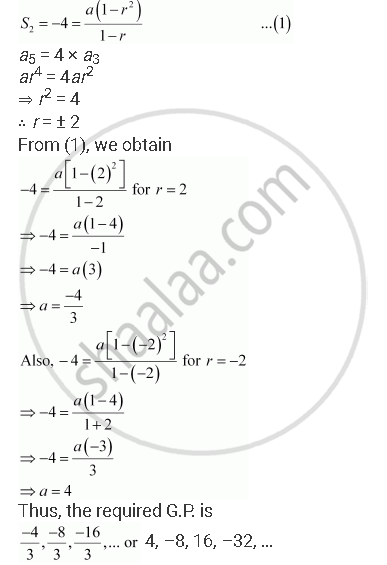CBSE (Arts) Class 11CBSE
Share

# Find a G.P. for Which Sum of the First Two Terms is –4 and the Fifth Term is 4 Times the Third Term. - CBSE (Arts) Class 11 - Mathematics

ConceptGeometric Progression (G. P.)

#### Question

Find a G.P. for which sum of the first two terms is –4 and the fifth term is 4 times the third term.

#### Solution

Let a be the first term and r be the common ratio of the G.P.

According to the given conditions,Is there an error in this question or solution?

#### APPEARS IN

NCERT Solution for Mathematics Textbook for Class 11 (2018 to Current)
Chapter 9: Sequences and Series
Q: 16 | Page no. 192

#### Video TutorialsVIEW ALL 

Solution Find a G.P. for Which Sum of the First Two Terms is –4 and the Fifth Term is 4 Times the Third Term. Concept: Geometric Progression (G. P.).
S